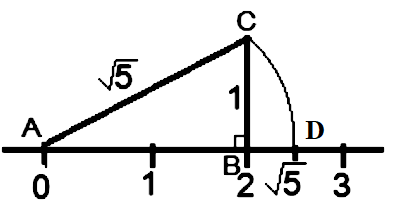Home/Class 9/Maths/

## QuestionMathsClass 9

Show how $$\sqrt {5}$$ can be represented on the number line.

See solution below
4.64.6## Solution

Firstly, draw a line segment $$AB$$ of length $$2$$ unit on the number line.
Then draw a perpendicular line segment $$(BC)$$ at point $$B$$ of $$1$$ unit.
Then join the points $$\mathrm{C}$$ and $$\mathrm{A}$$, to form a line segment $$(AC).$$
According to Pythagoras Theorem,
$$\Rightarrow A C^{2}=A B^{2}+B C^{2}$$
$$\Rightarrow A C^{2}=2^{2}+1^{2}$$
$$\Rightarrow A C=\sqrt{2^{2}+1^{2}}$$
$$\Rightarrow A C=\sqrt{5}$$
Now, draw the arc $$(ACD)$$, to get the number on the number line.